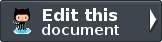# Key derivation function¶

In cryptography, a key derivation function (or KDF) derives one or more secret keys from a secret value such as a master key or other known information such as a password or passphrase using a pseudo-random function. For instance, a KDF function can be used to generate encryption or authentication keys from a user password. The Zend\Crypt\Key\Derivation implements a key derivation function using specific adapters.

User passwords are not really suitable to be used as keys in cryptographic algorithms, since users normally choose keys they can write on keyboard. These passwords use only 6 to 7 bits per character (or less). It is highly recommended to use always a KDF function of transformation a user’s password to a cryptography key.

Pbkdf2 is a KDF that applies a pseudorandom function, such as a cryptographic hash, to the input password or passphrase along with a salt value and repeats the process many times to produce a derived key, which can then be used as a cryptographic key in subsequent operations. The added computational work makes password cracking much more difficult, and is known as key stretching.

In the example below we show a typical usage of the Pbkdf2 adapter.

 ```1 2 3 4 5 6 7 8 9``` ```use Zend\Crypt\Key\Derivation\Pbkdf2; use Zend\Math\Rand; \$pass = 'password'; \$salt = Rand::getBytes(strlen(\$pass), true); \$key = Pbkdf2::calc('sha256', \$pass, \$salt, 10000, strlen(\$pass)*2); echo "Original password: \$pass \n"; echo "Key derivation : \$key \n"; ```

The Pbkdf2 adapter takes the password (\$pass) and generate a binary key with a size double of the password. The syntax is calc(\$hash, \$pass, \$salt, \$iterations, \$length) where \$hash is the name of the hash function to use, \$pass is the password, \$salt is a pseudo random value, \$iterations is the number of iterations of the algorithm and \$length is the size of the key to be generated. We used the Rand::getBytes function of the Zend\Math\Rand class to generate a random bytes using a strong generators (the true value means the usage of strong generators).

The number of iterations is a very important parameter for the security of the algorithm. Big values means more security. There is not a fixed value for that because the number of iterations depends on the CPU power. You should always choose a number of iteration that prevent brute force attacks. For instance, a value of 1‘000‘000 iterations, that is equal to 1 sec of elaboration for the PBKDF2 algorithm, is enough secure using an Intel Core i5-2500 CPU at 3.3 Ghz.

The SaltedS2k algorithm uses an hash function and a salt to generate a key based on a user’s password. This algorithm doesn’t use a parameter that specify the number of iterations and for that reason it’s considered less secure compared with Pbkdf2. We suggest to use the SaltedS2k algorithm only if you really need it.

Below is reported a usage example of the SaltedS2k adapter.

 ```1 2 3 4 5 6 7 8 9``` ```use Zend\Crypt\Key\Derivation\SaltedS2k; use Zend\Math\Rand; \$pass = 'password'; \$salt = Rand::getBytes(strlen(\$pass), true); \$key = SaltedS2k::calc('sha256', \$pass, \$salt, strlen(\$pass)*2); echo "Original password: \$pass \n"; echo "Key derivation : \$key \n"; ```

### Project Versions

#### Previous topic

Encrypt/decrypt using block ciphers

#### Next topic